• 叉积的计算是线段方法的核心。考虑如图33-1(a)所示的向量p1和p2。我们可以把叉积解释为由点(0,0)，p1，p2 和 p1+p2=(x1+x2,y1+y2)所构成的平行四边形的有向面积。另一种与之等价但更有效的叉积定义方式是将之看做...
叉积的计算是线段方法的核心。考虑如图33-1(a)所示的向量p1和p2。我们可以把叉积解释为由点(0,0)，p1，p2 和 p1+p2=(x1+x2,y1+y2)所构成的平行四边形的有向面积。另一种与之等价但更有效的叉积定义方式是将之看做矩阵行列式:

p1×p2=det[x1y1x2y2]=x1y2x2y1=p2×p1

p1×p2值为正,则相对于原点(0,0)来说，p1位于p2的顺时针方向；若 p1×p2值为负，则p1位于p2的逆时针方向。图(b)展示了向量p的顺时针和逆时针区域。叉积为0时出现边界情况；在这种情况下，两个向量是共线的，指向相同方向或者相反方向。为了确定相对于公共端点p0，有向线段p0p1是在顺时针还是逆时针方向更接近p0p2，我们将p0作为原点从而使问题简化。用p1-p0来表示向量p1=(x1,y1)，其中x1=x1x0y1=y1y0，类似的，可以定义p2-p0。然后，计算叉积

(p1p0)×(p2p0)=(x1x0)(y2y0)(x2x0)(y1y0)

下面是叉积的用途：

1.叉积判断线段拐向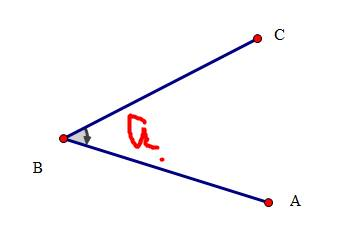如上图，可以通过计算向量的叉积来判断拐向，即判断（C-B）×（A-B）的符号。
#若（C-B）×（A-B）> 0 ，则CB在B点顺时针得到AB
#若（C-B）×（A-B）< 0 ，则CB在B点逆时针得到AB
#若（C-B）×（A-B）= 0 ，则A，B，C三点共线。

如图，我们给三个点A,B,C,判断沿着AB到BC，在B点是向右拐还是向左拐。并求出拐的 角度。这个先直接用叉积判断拐向，然后再余弦定理计算角度即可，
用180减去这个角度便是拐的角度。

2.叉积还有一个重要的用处，就是用来求面积

叉积用来求面积，求出的是有向面积，也就是说带正负号，
不过再取一下绝对值就是真正的面积大小了。
比如求三角形ABC的面积，先计算出叉积cross(A,B,C)，
那么面积就是fabs（cross(A,B,C)）/ 2.0
为什么呢？
我们看一下叉积的定义就知道了，a与b的叉积模为：
|a×b|=|a| |b| sin(a,b)他的几何意义就是以a和b为邻边的平行四边形的面积。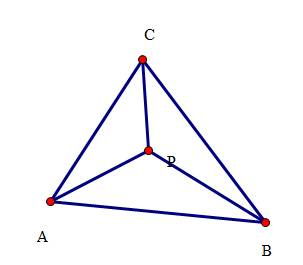3判断点p是否在三角形内。
若在三角形内，则必有S.abc=S.abp+S.acp+S.bcp;若在三角形外，式子就不成立。

4.叉积能判断两直线是否相交。

对于给出的两条线段A1A2，B1B2，判断他们是否相交需要分两步
（1）快速排斥实验
设以线段A1A2和线段B1B2为对角线的矩形为M,N;
若M,N 不相交，则两个线段显然不相交；
判断矩形相交，只需判断某一矩形是否有顶点在另一个矩形内即可。
所以：只有当满足第一个条件时，两个线段才可能相交。
（2）跨立实验
如果两线段相交，则两线段必然相互跨立对方.若A1A2跨立B1B2，则矢量( A1 - B1 ) 和(A2-B1)位于矢量(B2-B1)的两侧，
即：（(A1-B1) × (B2-B1) ）·（ (A2-B1) × (B2-B1)）<0。
上式可以改写成：（(A1-B1) × (B2-B1)） · （(B2-B1) × (A2-B1)）>0。
当(A1-B1) × (B2-B1)=0时，说明A1B1和B2B1共线，但前面已经通过了快速排斥实验，所以A1一定在B1B2上，所以判断线段A1A2是否跨立B1B2的依据就是：
（(A1-B1) × (B2-B1)） · （(B2-B1) × (A2-B1)）>=0。
同理，判断线段B1B2是否跨立A1A2的依据是：（(B1-A1) × (A2-A1)） · （(A2-A1) × (B2-A1)）>=0。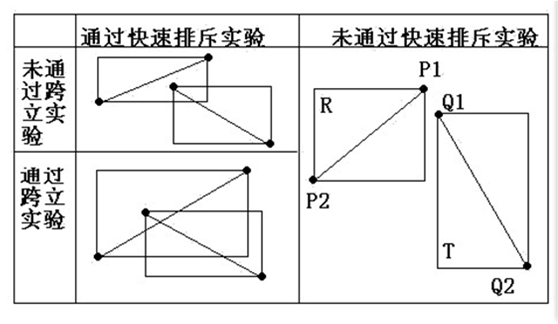展开全文codeswarrior 2018-04-06 16:04:03
• 向量叉积定义的证明 叉积的证明

后来打算写一篇叉积的证明时，却发现有些东西真的不好理解。设两个向量$\mathbf{a} = (x_1, y_1, z_1), \mathbf{b} = (x_2, y_2, z_2)$，两向量夹角为$\theta$，很多教材包括维基百科(Cross Product)等给出的定义都...

前面写了一篇向量点积定义的证明，由于这个证明比较简单，所以也没有引起深入的思考。后来打算写一篇叉积的证明时，却发现有些东西真的不好理解。

设两个向量$\mathbf{a} = (x_1, y_1, z_1), \mathbf{b} = (x_2, y_2, z_2)$，两向量夹角为$\theta$，很多教材包括维基百科(Cross Product)等给出的定义都是：

$$\mathbf{c} = \mathbf{a} \times \mathbf{b} = \mathbf{n} |\mathbf{a}| |\mathbf{b}| \sin{\theta}$$

其中$\mathbf{n}$是垂直于向量$\mathbf{a},\mathbf{b}$的单位向量，方向由右手法则确定。这样定义似乎没什么不妥，但是我在考虑一些问题：给出这个定义的数学家，他是怎么发现叉积的结果垂直于两向量？向量的模长为什么恰好等于$|\mathbf{a}| |\mathbf{b}| \sin{\theta}$？下面给出我对这些问题的理解。

我想数学家们刚开始定义向量的叉乘运算($\times$)时，给出的唯一基本定义是：$\mathbf{a} \times \mathbf{b}$的结果$\mathbf{c}$是垂直于向量$\mathbf{a},\mathbf{b}$的一个向量，其方向由右手法则确定；如果向量$\mathbf{a},\mathbf{b}$平行，则叉积结果为零向量。有了这个定义，再根据乘法对加法的分配率，便可得到叉积运算的坐标表达式：

\begin{align} \mathbf{a} \times \mathbf{b} = & (x_1\mathbf{i} + y_1\mathbf{j} + z_1\mathbf{k}) \times (x_2\mathbf{i} + y_2\mathbf{j} + z_2\mathbf{k}) \\ = &\ x_1\mathbf{i} \times (x_2\mathbf{i} + y_2\mathbf{j} + z_2\mathbf{k}) + y_1\mathbf{j} \times (x_2\mathbf{i} + y_2\mathbf{j} + z_2\mathbf{k}) + z_1\mathbf{k} \times (x_2\mathbf{i} + y_2\mathbf{j} + z_2\mathbf{k}) \\ = &\ x_1 x_2 (\mathbf{i} \times \mathbf{i}) + x_1 y_2 (\mathbf{i} \times \mathbf{j}) + x_1 z_2 (\mathbf{i} \times \mathbf{k}) + \\ &\ y_1 x_2 (\mathbf{j} \times \mathbf{i}) + y_1 y_2 (\mathbf{j} \times \mathbf{j}) + y_1 z_2 (\mathbf{j} \times \mathbf{k}) + \\ &\ z_1 x_2 (\mathbf{k} \times \mathbf{i}) + z_1 y_2 (\mathbf{k} \times \mathbf{j}) + z_1 z_2 (\mathbf{k} \times \mathbf{k}) \end{align}

其中$\mathbf{i}, \mathbf{j}, \mathbf{k}$分别表示x、y、z轴方向的单位向量。那么根据向量叉积的定义：$\mathbf{i} \times \mathbf{i} = \mathbf{j} \times \mathbf{j} = \mathbf{k} \times \mathbf{k} = \mathbf{0}$，$\mathbf{i} \times \mathbf{j} = \mathbf{k}$，$\mathbf{j} \times \mathbf{k} = \mathbf{i}$，$\mathbf{k} \times \mathbf{i} = \mathbf{j}$，$\mathbf{j} \times \mathbf{i} = -\mathbf{k}$，$\mathbf{k} \times \mathbf{j} = -\mathbf{i}$，$\mathbf{i} \times \mathbf{k} = -\mathbf{j}$，因此便得到：

\begin{align} \mathbf{a} \times \mathbf{b} &= (y_1 z_2 - z_1 y_2) \mathbf{i} + (z_1 x_2 - x_1 z_2) \mathbf{j} + (x_1 y_2 - y_1 x_2) \mathbf{k} \\ &= (y_1 z_2 - z_1 y_2, \ z_1 x_2 - x_1 z_2, \ x_1 y_2 - y_1 x_2)\end{align}

下面来证明$|\mathbf{c}| = |\mathbf{a}| |\mathbf{b}| \sin{\theta}$：

\begin{align} |\mathbf{c}|^2 = &\ (y_1 z_2 - z_1 y_2)^2 + (z_1 x_2 - x_1 z_2)^2 + (x_1 y_2 - y_1 x_2)^2 \\ = &\ y_1^2 z_2^2 + z_1^2 y_2^2 - 2y_1 y_2 z_1 z_2 + z_1^2 x_2^2 + x_1^2 z_2^2 - 2x_1 x_2 z_1 z_2 + \\ &\ x_1^2 y_2^2 + y_1^2 x_2^2 - 2x_1 x_2 y_1 y_2 \end{align}

又根据向量点积的定义：

\begin{align} (|\mathbf{a}| |\mathbf{b}| \sin{\theta})^2 &= (|\mathbf{a}| |\mathbf{b}|)^2 \sin^{2}{\theta} \\ &= (|\mathbf{a}| |\mathbf{b}|)^2 (1 - \cos^{2}{\theta}) \\ &= (|\mathbf{a}| |\mathbf{b}|)^2 \left(1 - \frac{(\mathbf{a} \cdot \mathbf{b})^2}{(|\mathbf{a}| |\mathbf{b}|)^2} \right) \\ &=  (|\mathbf{a}| |\mathbf{b}|)^2 - (\mathbf{a} \cdot \mathbf{b})^2 \end{align}

因为：

\begin{align} (|\mathbf{a}| |\mathbf{b}|)^2 = &\ \left( \sqrt{x_1^2 + y_1^2 + z_1^2} \sqrt{x_2^2 + y_2^2 + z_2^2} \right)^2 \\ = &\ (x_1^2 + y_1^2 + z_1^2) (x_2^2 + y_2^2 + z_2^2) \\ = &\ x_1^2 x_2^2 + y_1^2 y_2^2 + z_1^2 z_2^2 + x_1^2 y_2^2 + x_1^2 z_2^2 + y_1^2 x_2^2 + y_1^2 z_2^2 + z_1^2 x_2^2 + z_1^2 y_2^2 \end{align}

而且

\begin{align} (\mathbf{a} \cdot \mathbf{b})^2 = &\ (x_1 x_2 + y_1 y_2 + z_1 z_2)^2 \\ = &\ x_1^2 x_2^2 + y_1^2 y_2^2 + z_1^2 z_2^2 + 2x_1 x_2 y_1 y_2 + 2x_1 x_2 z_1 z_2 + 2y_1 y_2 z_1 z_2 \end{align}

容易看出：$(|\mathbf{a}| |\mathbf{b}|)^2 - (\mathbf{a} \cdot \mathbf{b})^2 = |\mathbf{c}|^2$，即：

$$|\mathbf{c}| = |\mathbf{a}| |\mathbf{b}| \sin{\theta}$$

展开全文weixin_39815345 2020-12-19 07:57:23
• 点积和叉积在计算机图形学的应用 线性代数 叉积 向量

点积和叉积在计算机图形学中，是最为基础且重要的概念，初学者弄清它的概念的应用，是很重要的。 先说明下，以下均采用列向量的表示方法，和线性代数书本上的行向量不同，采用列向量表示，则表达为列向量左乘矩阵，...

点积和叉积在计算机图形学中，是最为基础且重要的概念，初学者弄清它的概念的应用，是很重要的。最后一节，是为了加强理解记录，如果不看也是可以的，大家选择观看，有兴趣可以去看原视频，结合我的笔记。

前置知识

1. 列向量
以下均采用列向量的表示方法，和线性代数书本上的行向量不同，采用列向量表示，则表达为列向量左乘矩阵，只是定义的不同，其他含义没有什么不同。列向量写法如下：
a → = ( x y z ) \overrightarrow{a} = \left( \begin{gathered} \begin{matrix} x \\ y \\ z \end{matrix} \end{gathered} \right)

2. 单位化
将向量的各分量除以向量的模即得单位向量，单位指模为1的向量。

3. 向量加减法的几何意义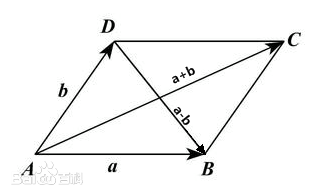4. 行列式
行列式在数学中，是一个函数，其定义域为det的矩阵A，取值为一个标量，写作det(A)或 | A | 。行列式可以看做是有向面积（二维）或体积（三维）的概念在一般的欧几里得空间中的推广。

点积

点积在数学中，又称数量积（dot product; scalar product），是指接受在实数R上的两个向量并返回一个实数值标量的二元运算。它是欧几里得空间的标准内积。点积的结果是一个数。

a → ⋅ b → = ∣ a ∣ ∣ b ∣ c o s θ \overrightarrow{a} \cdot \overrightarrow{b} = \left| a \right| \left| b \right| cos {\theta}

相当于a向量的转置矩阵乘以向量b：
a → ⋅ b → = a → T b → \overrightarrow{a} \cdot \overrightarrow{b} = \overrightarrow{a}^T \overrightarrow{b}

以二维向量为例：
a → ⋅ b → = ( x a y a ) T ( x a y b ) = x a x b + y a y b \overrightarrow{a} \cdot \overrightarrow{b} = {x_{a}\choose y_{a}}^T {x_{a}\choose y_{b}} = x_{a}x_{b} + y_{a}y_{b}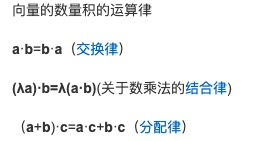几何意义a向量在b向量上投影的长度 乘以 b向量的长度（模）就是 点积的结果。
点乘是存在交换律的，所以也等同于：
b向量在a向量上投影的长度 乘以 a向量的长度就是 点积的结果。

在图形学的应用

1. 求两个向量的夹角
a → \overrightarrow{a} b → \overrightarrow{b} 都是单位向量（单位化），两个向量点积就是 cos ⁡ θ \cos {\theta} ，在通过 arccos ⁡ \arccos 就可以求出夹角。

2. 求投影
a → \overrightarrow{a} 单位化， a → ⋅ b → \overrightarrow{a} \cdot \overrightarrow{b} 就是 b → \overrightarrow{b} a → \overrightarrow{a} 上的投影长度。
《计算机图形学》书上有这么一个举例，利用投影将向量w分解成两个向量和：3. 比较两个向量的接近程度（方向上）
也就是向量是否两个向量间的夹角大小，在聚光灯的效果计算中，可以根据点积来得到光照效果，如果点积越大，说明夹角越小，则物体离光照的轴线越近，光照越强。
也常会用来判断两向量是否为同方向：举个例子

判断一个点是都在某图形内：叉积

向量积，数学中又称外积、叉积，物理中称矢积、叉乘，是一种在向量空间中向量的二元运算。与点积不同，它的运算结果是一个向量而不是一个标量。并且两个向量的叉积与这两个向量和垂直。
∣ v → × w → ∣ = ∣ v ∣ ∣ w ∣ s i n θ \left| \overrightarrow{v} \times \overrightarrow{w} \right| = \left| v \right| \left| w \right| sin {\theta}
这只确定了叉积的长度，众所周知向量有长度，还有方向的，方向为垂直于两个向量的方向，但是呢，垂直方向有两个。
这里我们采用右手螺旋定则确定方向。v到w的方向就是右手四指弯曲的方向，大拇指的方向就是叉积的方向。二维向量的叉积是个标量，看起来违背了定义，先假设二维向量得出来的叉积是z，如果把二维向量看作成z轴值恒为0的三维向量，它们的叉积就 ( 0 , 0 , z ) T (0,0,z)^T ，三维空间被压缩成了二维，叉积就只是一个标量了。

v → ⋅ w → = x v y w − y v x w \overrightarrow{v} \cdot \overrightarrow{w} = x_vy_w - y_vx_w

三维向量的叉积的结果是个向量：

v → ⋅ w → = ( y v z w − z v y w z v x w − x v z w x v y w − y v x w ) \overrightarrow{v} \cdot \overrightarrow{w} = \left( \begin{gathered} \begin{matrix} y_v z_w - z_v y_w \\ z_vx_w - x_vz_w \\ x_vy_w - y_vx_w \end{matrix} \end{gathered} \right)
这个看起来很难记忆，我们随后再讲解。444额方法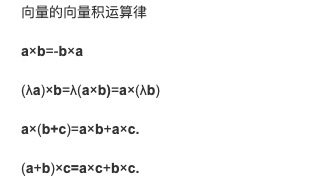几何意义

在二维空间，两个向量叉积就是两向量形成的平行四边形的面积，正负要引入Z轴，由右手螺旋定则确定，如果大拇指指向Z正轴就为正，反之为负；
在三维空间，两个向量叉积就是垂直于两向量形成的平面的向量（称为法向量）采用右手螺旋定则确定方向。v到w的方向就是右手四指弯曲的方向，大拇指的方向就是叉积的方向。

坐标系的问题

如果我们已知x坐标轴和y坐标轴，可以推导出z坐标轴。可能大家都听说过有左手坐标系和右手坐标系这两种说法，可以用x、y轴叉积与z轴的关系确定。
右手坐标系：
x → × y → = z → \overrightarrow{x} \times \overrightarrow{y} = \overrightarrow{z}
左手坐标系：
x → × y → = − z → \overrightarrow{x} \times \overrightarrow{y} = -\overrightarrow{z}

在图形学的应用

1. 二维空间判断左右这个判断又可以衍生出如何判断一条路径存在交叉
2. 三维空间求法向量
法向量在计算图形学的应用很广泛，很多地方都会利用到，比如求已知入射角度 O B → \overrightarrow{OB} 求一个平面的反射角度 B P → \overrightarrow{BP} 。取平面上不共线的三个点ABC，求得法线
A B → × A C → = Z → （ 法 线 ） \overrightarrow{AB} \times \overrightarrow{AC} = \overrightarrow{Z} （法线）B P → = O B → − Z → \overrightarrow{BP} = \overrightarrow{OB} - \overrightarrow{Z}

再举个例子

判断一个点是都在某图形内以线性变换去看点乘和叉乘

这部分我是基于B站的一部分的视频总结的，如果觉得作者讲解不够好，可以去看原视频

点乘

先来看两向量的点乘另一种求法，a向量的转置矩阵乘以向量b：
a → ⋅ b → = a → T b → \overrightarrow{a} \cdot \overrightarrow{b} = \overrightarrow{a}^T \overrightarrow{b}
以二维空间为例，定义两个基向量组成的矩阵( 2 1 ) = 2 i → + j → \left( \begin{array}{c} 2 \\ 1 \end{array} \right)= 2\overrightarrow{i} + \overrightarrow{j}
点乘就是从二维空间到数轴的线性变换，将空间投影到给定的数轴来定义。
a → T \overrightarrow{a}^T 矩阵作用就是，将基变量在 a → \overrightarrow{a} 上投影为两个数字，而不是向量，将此作为基变量去做线性变换，从而进行空间压缩。

叉乘

截了视频的两张图，[ x y z ] \left[ \begin{array}{c} x \\ y \\ z\end{array} \right] 表示一个随机变量，也可理解为一个为向量的变量。
上图是一个变量为向量的函数。根据v w 可以求常量 p有没有觉得 p → \overrightarrow{p} u → × v → \overrightarrow{u} \times \overrightarrow{v} 叉积一模一样，那么我们认定 p → \overrightarrow{p} 就是 u → × v → \overrightarrow{u} \times \overrightarrow{v} 的 叉积，来解释叉积的几何意义。

先看左边， p → \overrightarrow{p} 与随机变量的点积，其实就随机变量在 p → \overrightarrow{p} 的投影长度，再乘以 p → \overrightarrow{p} 的模。（1）

再看看右边，是一个行列式，表示这三个向量组成的立方体的体积大小。（2）

我们再回顾下，体积的求法，高✖️底面积（u v 组成底面）（3）

高为随机变量在平面垂直方向的投影长度。（4）

由（2）（3）（4）得到右边为 随机变量在平面垂直方向的投影长度，再乘以u v 组成的平面面积（5）

由（1）（5）得到， p → \overrightarrow{p} 为，模长为两向量组成的平面面积，且为这个平面的法线。

这个叉积的几何意义不就是这样的嘛。

字丑的那些图都是我画的，除了一张小手手，请各位见谅，最后感谢我的首席插画师 yc君 画的那种小手手的插图，O(∩_∩)O哈哈~。

展开全文from_the_star 2020-05-29 23:05:20
• 在3D游戏开发中，经常用到向量的点积和叉积及其几何意义，为防止遗忘，在此记录一下。点积在数学中，点积(德语：Skalarprodukt、英语：Dot Product)又称数量积或标量积(德语：Skalarprodukt、英语：Scalar Product)...

在3D游戏开发中，经常用到向量的点积和叉积及其几何意义，为防止遗忘，在此记录一下。

点积

在数学中，点积(德语：Skalarprodukt、英语：Dot Product)又称数量积或标量积(德语：Skalarprodukt、英语：Scalar Product)，是一种接受两个等长的数字序列(通常是坐标向量)、返回单个数字的代数运算。在欧几里得几何中，两个笛卡尔坐标向量的点积常称为内积(德语：inneres Produkt、英语：Inner Product)，见内积空间。

定义

点积有两种定义方式：代数方式和几何方式。通过在欧氏空间中引入笛卡尔坐标系，向量之间的点积既可以由向量坐标的代数运算得出，也可以通过引入两个向量的长度和角度等几何概念来求解。

代数定义

两个向量 $\vec{a} = [a1, a2,…, an]$和 $\vec{b} = [b1, b2,…, bn]$的点积定义为：

$$\vec{a}\cdot \vec{b} = \sum_{i=1}^n a_ib_i = a_1b_1 + a_2b_2 + \cdots + a_nb_n$$

这里的Σ是求和符号，而n是向量空间的维数。

几何定义

在欧几里得空间中，点积可以直观地定义为

$$\vec{a} \cdot \vec{b} = |\vec{a}| , |\vec{b}| \cos \theta ;$$

这里 $|\vec{x}|$ 表示 $\vec{x}$的模(长度)， $\theta$ 表示两个向量之间的角度。

叉积

在数学和向量代数领域，叉积(英语：Cross product)又称向量积(英语：Vector product)，是对三维空间中的两个向量的二元运算，使用符号 $\times$。与点积不同，它的运算结果是向量。对于线性无关的两个向量 $\mathbf {a}$ 和 $\mathbf {b}$ ，它们的叉积写作 ${\mathbf {a} \times \mathbf {b} }$，是 $\mathbf {a}$ 和 $\mathbf {b}$ 所在平面的法线向量，与 $\mathbf {a}$ 和 $\mathbf {b}$ 都垂直。叉积被广泛运用于数学、物理、工程学、计算机科学领域。

定义

两个向量 $\mathbf {a}$ 和 $\mathbf {b}$ 的叉积仅在三维空间中有定义，写作 $\mathbf {a} \times \mathbf {b}$。在物理学中，叉积有时也被写成$\mathbf {a} \wedge \mathbf {b}$，但在数学中 $\mathbf {a} \wedge \mathbf {b}$ 是外代数中的外积。

叉积 $\mathbf {a} \times \mathbf {b}$ 是与 $\mathbf {a}$ 和 $\mathbf {b}$ 都垂直的向量 $\mathbf {c}$ 。其方向由右手定则决定，模长等于以两个向量为边的平行四边形的面积。叉积可以定义为：

$$\mathbf {a} \times \mathbf {b} =|\mathbf {a} ||\mathbf {b} |\sin(\theta )\ \mathbf {n}$$

其中$\theta$ 表示 $\mathbf {a}$ 和 $\mathbf {b}$ 在它们所定义的平面上的夹角( $0^{\circ }\leq \theta \leq 180^{\circ }$)。 $|\mathbf {a} |$ 和 $|\mathbf {b} |$ 是向量$\mathbf {a}$ 和 $\mathbf {b}$ 的模长，而 $\mathbf{n}$ 则是一个与 $\mathbf {a}$ 、 $\mathbf {b}$ 所构成的平面垂直的单位向量，方向由右手定则决定。根据上述公式，当$\mathbf {a}$ 与 $\mathbf {b}$ 平行(即 $\theta$ 为 0° 或 180°)时，它们的叉积为零向量 $\mathbf{0}$。矩阵表示

叉积可以表达为这样的行列式：

$$\mathbf {u\times v} ={\begin{vmatrix}\mathbf {i} &\mathbf {j} &\mathbf {k} \u_{1}&u_{2}&u_{3}\v_{1}&v_{2}&v_{3}\\end{vmatrix}}$$

这个行列式可以使用萨吕法则或拉普拉斯展开计算。使用拉普拉斯展开可以沿第一行展开为：

{\begin{aligned}\mathbf {u\times v} &={\begin{vmatrix}u_{2}&u_{3}\v_{2}&v_{3}\end{vmatrix}}\mathbf {i} -{\begin{vmatrix}u_{1}&u_{3}\v_{1}&v_{3}\end{vmatrix}}\mathbf {j} +{\begin{vmatrix}u_{1}&u_{2}\v_{1}&v_{2}\end{vmatrix}}\mathbf {k} \&=(u_{2}v_{3}-u_{3}v_{2})\mathbf {i} -(u_{1}v_{3}-u_{3}v_{1})\mathbf {j} +(u_{1}v_{2}-u_{2}v_{1})\mathbf {k} \end{aligned}}

可以直接得到结果向量。

参考链接叉积, by wikipedia.

数量积, by wikipedia.

展开全文weixin_39612877 2020-12-20 17:54:52
• zsyzClb 2019-05-11 10:02:41
• 向量叉积的应用（求多边形面积、凸包问题） 经验分享

Shanhj 2021-09-24 14:40:26
• weixin_39584571 2020-11-02 15:42:07
• weixin_39885803 2020-11-02 09:33:31
• 凸包问题（含叉积讲解） 算法 acm竞赛

qq_50966565 2021-08-01 11:40:29
• shk0010 2019-09-24 18:47:30
• Unity 旋转矩形的区域检测（向量叉积算法实现） unity

a95537709 2021-07-16 17:15:08
• 计算几何基础——矢量和叉积 && 叉积、线段相交判断、凸包（转载） 两直线平行交叉相乘

weixin_39628384 2020-12-19 23:10:51
• 线性代数-叉积 线性代数

weixin_41479678 2021-05-12 10:55:33
• 线性代数【19】叉积 线性代数 算法 矩阵

yellow_hill 2021-12-07 16:30:07
• 向量叉积分配律简单证明 叉积 向量 线性代数

qq_39565901 2020-10-13 20:53:16
• 向量叉积与向量叉积的模的运算 算法 vb.net

qq_38610729 2020-10-09 10:11:03
• it_xiangqiang 2021-03-18 11:20:51
• 线段相交 叉积 线段相交

lily1234567 2019-02-27 16:27:12
• weixin_35761503 2020-12-30 09:40:34...